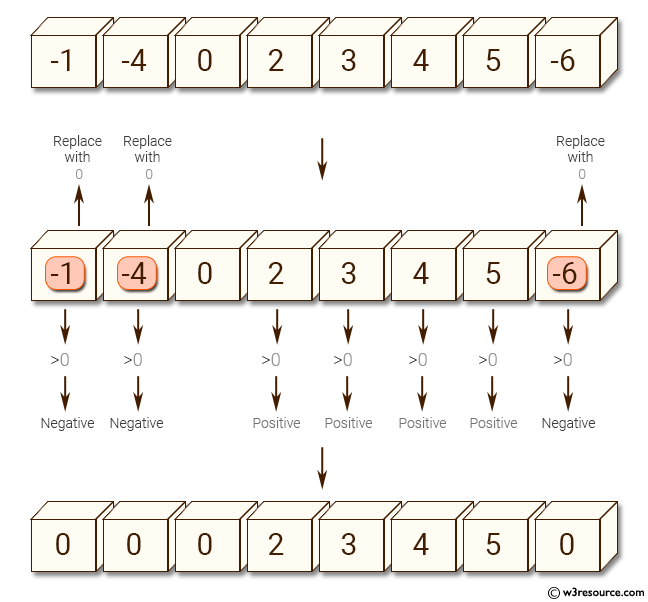﻿ NumPy: Replace the negative values in a NumPy array with 0 - w3resource# NumPy: Replace the negative values in a NumPy array with 0

## NumPy: Array Object Exercise-90 with Solution

Write a NumPy program to replace the negative values in a NumPy array with 0.

Pictorial Presentation:Sample Solution:

Python Code:

``````import numpy as np
x = np.array([-1, -4, 0, 2, 3, 4, 5, -6])
print("Original array:")
print(x)
print("Replace the negative values of the said array with 0:")
x[x < 0] = 0
print(x)
```
```

Sample Output:

```Original array:
[-1 -4  0  2  3  4  5 -6]
Replace the negative values of the said array with 0:
[0 0 0 2 3 4 5 0]
```

Python Code Editor:

Have another way to solve this solution? Contribute your code (and comments) through Disqus.

What is the difficulty level of this exercise?

Test your Python skills with w3resource's quiz

﻿

## Python: Tips of the Day

List comprehension:

```>>> m = [x ** 2 for x in range(5)]
>>> m
[0, 1, 4, 9, 16]
```